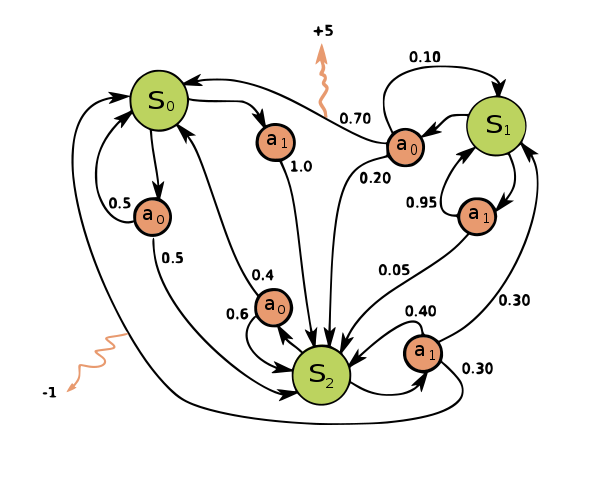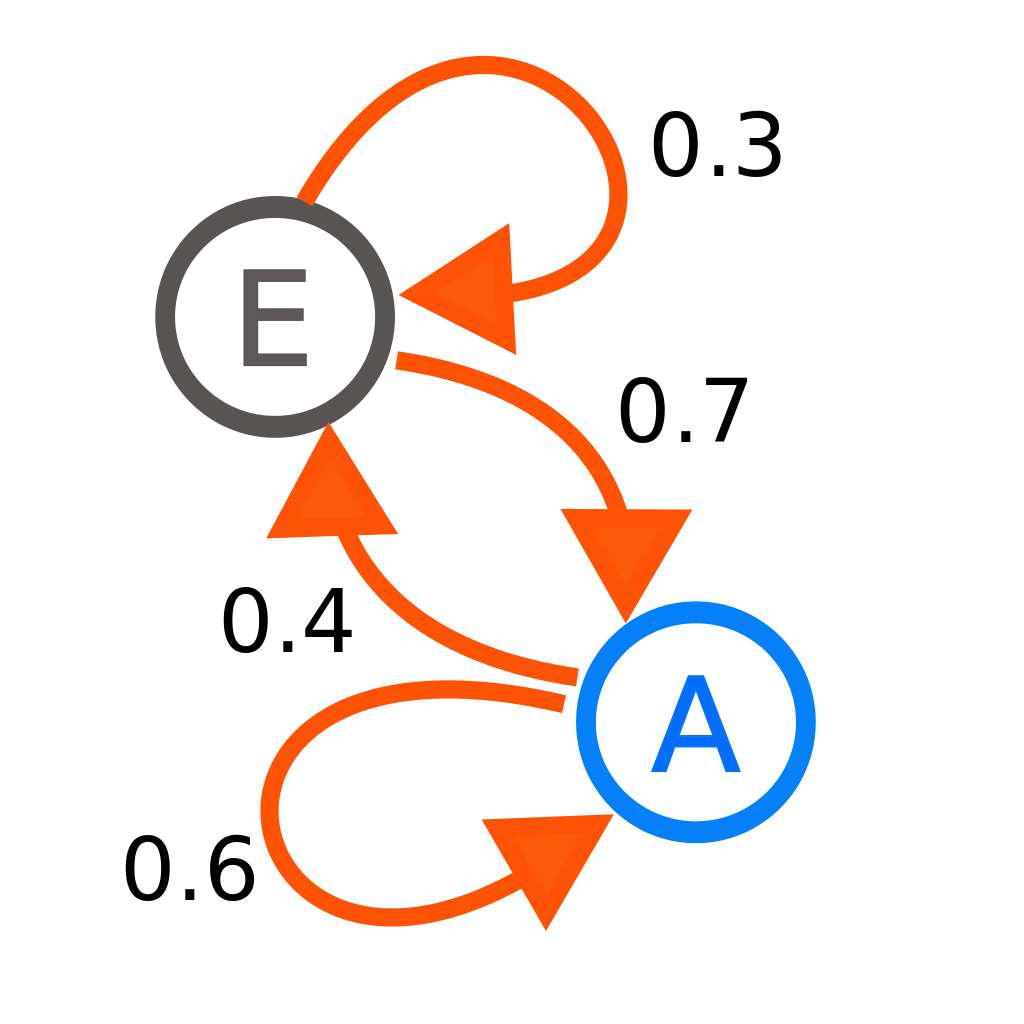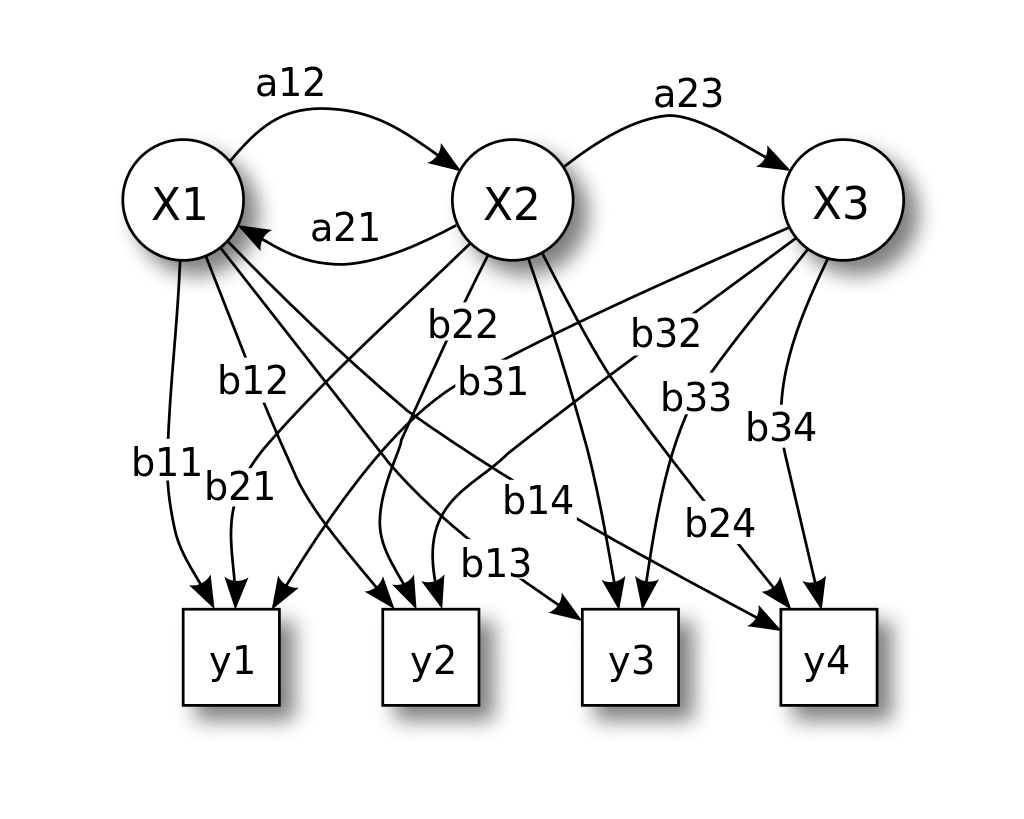# 强化学习102 - 马尔科夫决策过程（Markov Decision Process）的学习笔记## 1 引入

A stochastic process has the Markov property if the conditional probability distribution of future states of the process (conditional on both past and present states) depends only upon the present state, not on the sequence of events that preceded it.### 1.1 马尔科夫链 Markov Chain### 1.2 隐马尔科夫模型 Hidden Markov Model，HMM### 1.3 马尔科夫决策过程 Markov Decision Process## 2 马尔科夫决策过程 Markov Decision Process

star——星星？并不是，其实是我在学习过程中看到马尔科夫决策过程分别由$s,t,a,r$等基本元素组成。: ）

### 2.1 行动链条${\{s_0,a_0,s_1,a_1,...,s_{t-1},a_{t-1},a_t\}}$

1. 下一个状态是环境决定的
2. 下一个动作是小机器人的策略决定的

### 2.2 下一个动作$a_t^*=argmax_{a_{t_i}}p(a_{t_i}|{\{s_0,a_0,s_1,a_1,...,s_{t-1},a_{t-1},a_t\}})$

argmax表示取使函数最大的自变量，星表示最优策略。$a_t^*=argmax_{a_{t_i}}p(a_i|{s_t})$

### 2.3 下一个状态$p(S_{t+1}|S_t,a_t)$

### 2.4 奖励、近视远视与贝尔曼方程$\sum{\gamma^t r_k}$$v_\pi$为依据回报得到的价值函数，那么根据我们之前设置的“远视”系数，可以表示为：$v_\pi(s_t)=\sum^{\infty}_{k=0}\gamma^t r_k$$v_\pi(s_t)=\sum^{\infty}_{k=0}\gamma^k r_k$$= r_t + \gamma\sum^{\infty}_{k=1}\gamma^{k-1} r_k$$= r_t + \gamma v_\pi(s_{t+1})$

## 3 总结

Subscribe9 评论
Inline Feedbacks6 月 之前

[…] 2018-12-26 强化学习102 – 马尔科夫决策过程（Markov Decision Process）的学习笔记 […]Alexander
2 年 之前3 年 之前3 年 之前yoghourt
3 年 之前